# F.3 Approximation of the Self-Energy

Depending on the problem, one can either attempt a summation of a selected class of dominant contributions or perform an expansion with respect to the screened interaction. As shown in Appendix F.1.1, the self-energy itself and the polarization propagator, which determines the screened interaction, depend sensitively on the vertex corrections. Thus there is a complicated functional dependence of single-particle properties on two-particle properties and vice versa, and one has to consider the consistence of approximations at the single-particle and two-particle levels. In principle, there are two different approaches to attack the coupled system of equations derived in Appendix F.1.1 An iterative procedure and self-consistent approximations.

Starting such an iterative solution, one can first neglect vertex corrections in (F.23) and obtain an approximation for the self-energy by means of (F.14) together with (F.21) and (F.22). Making use of this approximation, one calculates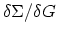, and includes vertex corrections in the next step. The iteration of such a procedure generates an expansion in terms of the screened interaction and the GREEN's function defined as a self-consistent solution of the DYSON equation.

For the iterative procedure, the sequence of steps can be defined by the vertex function (F.23), which yields by means of the chain rule the recurrence relation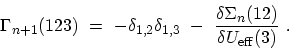(F.54)

One starts with the HARTREE-approximation, i.e., which delivers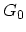,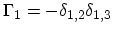and the screened interaction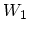. In the subsequent step one obtains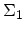,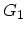and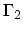and so forth. The effect of this interaction is two-fold. In the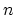-th step, the GREEN's functions contributing to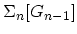become dressed by an additional interaction line and additionally new types of diagrams are generated.

For the Self-consistent approximations, one selects a certain class of self-energy diagrams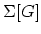. The DYSON equation becomes a non-linear functional equation of the GREEN's functions, which has to be solved self-consistently. The selection corresponds to the summation of a certain class of diagrams up to infinite-order in the interaction, whereas others which contribute even in lower order are neglected. The difficulty is in finding the correct way to choose a subset of diagrams for each order. In order to deliver physically meaningful results, any approximation should guarantee certain macroscopic conservation laws. This condition can be imposed by the postulate that all diagrams contributing to the self-energy are obtained from the functional derivative of a functional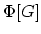with respect to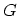. Solving the DYSON equation self-consistently with a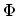-derivable self-energy yields a GREEN's function which conserves particle number, energy, and momentum .

M. Pourfath: Numerical Study of Quantum Transport in Carbon Nanotube-Based Transistors